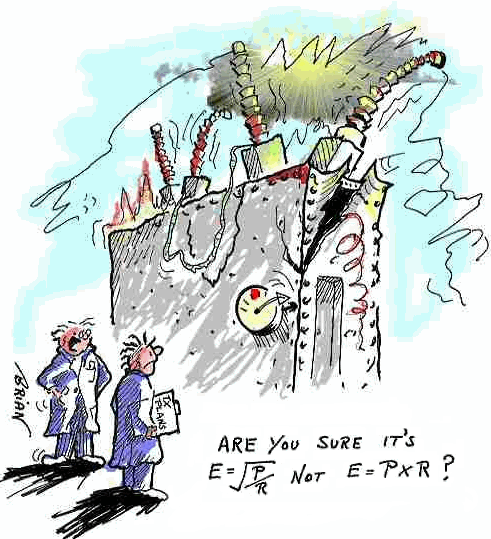## Power Triangle

### Magic Triangles

Electronics is a major part of some aerospace technician’s workday.  As an aerospace technician, it is considered a basic skill to be able to calculate voltage, current (amps), power (watts), and resistance (ohms), but remembering the formulas that go into those calculations can be difficult.  This is where a “magic triangle” can help.

The first thing you need to remember is the correct formula sign.  What letter or symbol represents each of these values.  The table below should help and can be cut out and kept in your badge holder if you want.

 Name Formula sign Unit Symbol voltage V or E volt V current I ampere (amp) A resistance R ohm Ω power P watt W

To calculate power (watts), voltage, and current you can try to memorize three different formulas depending on which value your looking for or you can use a Power Triangle.

To find the value your looking for, just cover up the formula sign on the triangle and do the calculation you see.  Let’s say you have measured the volts and current with your multimeter, you can cover the P up on the triangle with your thumb and see that you need to multiply volts by current to get the wattage (power) that is being passed over the line.  Or, say you want to find out the volts and all you can measure is the watts (P) and current (I).  You cover up the V on the triangle and discover you need to divide P by I to get the answer.  It’s really not that difficult using the Power Triangle and sure beats trying to remember three separate formulas.Credit sengpielaudio.com

To calculate resistance using the Ohms law, there is another magic triangle called the Ohms Law Triangle.  This triangle will help you calculate resistance, current (I), and voltage.  This can also help you figure out how large of a resistor you want to place in a circut to obtain a specified current and voltage.

To calculate what type of resistor you need to obtain the proper resistance in a circuit, you would cover the R on the triangle and see that you need to divide Volts by Current.  If you already have a resistor on the circuit and wish to know the current, you can divide volts by the resistance to get the answer after you cover up the R on the triangle.  Not too hard is it?

For more advanced calculations, you might also find this Power Wheel handy.

Overall, power calculations don’t have to keep you up at night or calling your former electronics instructor right in the middle of a job.  All it takes is just a couple “magic triangles” to help you through.

Friday, January 28th, 2011 Basic Electricity Comments Off on Magic Triangles